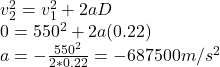## Meteorite On October 9, 1992, a 27-pound meteorite struck a car in Peekskill, NY, creating a dent about 22 cm deep. If the initial speed of

Question

Meteorite On October 9, 1992, a 27-pound meteorite struck a car in Peekskill, NY, creating a dent about 22 cm deep. If the initial speed of the meteorite was 550 m/s, what was the average force exerted on the meteorite by the car?

in progress 0
2 months 2021-07-28T08:58:43+00:00 2 Answers 1 views 0

The average force exerted on the meteorite by the car is 8.435×10⁶N

Explanation:

Given:

m = 27 lb = 12.27 kg

D = 22 cm = 0.22 m

v1 = 550 m/s

The acceleration of the meteorite is:Negative sign indicates opposite to the initial velocity. The average force is equal to:

Magnitude of a = 687500 m/s²

F = m*a = 12.27 * 687500 = 8.435×10⁶N

8427375 N

Explanation:

From newton’s equation of motion,

F = ma…………….. Equation 1

Where F = average force exerted on the meteorite by the car, m = mass of the meteorite, a = acceleration.

We can find the value of acceleration from newton’s equation of motion

using,

v² = u²+2as……………… Equation 2

Where v = final velocity, u = initial velocity, a = acceleration, s = distance.

Make a the subject of the equation

a = (v²-u²)/2s…………… Equation 3

Note: When the meteorite struck the car, it was brought to rest

Given: u = 550 m/s, v = 0 m/s ( brought to rest), s = 22 cm = 0.22 m

Substitute into equation 3

a = (0²-550²)/(2×0.22)

a = -302500/0.44

a = -687500 m/s²

Also given: m = 27 pound = (27×0.454) = 12.258 kg

Substitute into equation 1

F = 12.258(-687500)

F = -8427375 N

Note: The negative sign tells that the force act in opposite direction to the motion of the meteorite